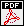## Coates-Wiles homomorphisms and Iwasawa cohomology for Lubin-Tate extensions

Autors: Peter Schneider and Otmar Venjakob
Titel:  Coates-Wiles homomorphisms and Iwasawa cohomology for Lubin-Tate extensions
Jahr: 2015
Seiten: 54
In: Elliptic curves, modular forms and Iwasawa theory, 401--468, Springer Proc. Math. Stat., 188, Springer, Cham, 2016.Abstract: For the $p$-cyclotomic tower of $\mathbb{Q}_p$ Fontaine established a description of local Iwasawa cohomology with coefficients in a local Galois representation $V$ in terms of the $\psi$-operator acting on the attached etale $(\varphi,\Gamma)$-module $D(V)$. In this article we generalize Fontaine's result to the case of arbitratry Lubin-Tate towers $L_\infty$ over finite extensions $L$ of $\mathbb{Q}_p$ by using the Kisin-Ren/Fontaine equivalence of categories between Galois representations and $(\varphi_L,\Gamma_L)$-module and extending parts of \cite{Her}, \cite{Sch}. Moreover, we prove a kind of explicit reciprocity law which calculates the Kummer map over $L_\infty$ for the multiplicative group twisted with the dual of the Tate module $T$ of the Lubin-Tate formal group in terms of Coleman power series and the attached $(\varphi_L,\Gamma_L)$-module. The proof is based on a generalized Schmid-Witt residue formula. Finally, we extend the explicit reciprocity law of Bloch and Kato \cite{BK} Thm.\ 2.1 to our situation expressing the Bloch-Kato exponential map for $L(\chi_{LT}^r)$ in terms of generalized Coates-Wiles homomorphisms, where the Lubin-Tate characater $\chi_{LT}$ describes the Galois action on $T.$